# G'MIC exercises

There is no miscount.

Try this. The \$31,\$32 has to be combined. For points or two variables representing one GUI element, combine them.

``````u "{\$1}"\
"{\$2}"_{\$1==4?2:0}\
"{\$3}"_{\$1==4?2:0}\
"{\$4}"_{\$1==4?2:0}\
"{\$5}"_{\$1==4?2:0}\
"{\$6}"\
"{\$7}"\
"{\$8}"\
"{\$9}"\
"{\$10}"\
"{\$11}"\
"{\$12}"\
"{\$13}"\
"{\$14}"\
"{\$15}"\
"{\$16}"\
"{\$17}"\
"{\$18}"\
"{\$19}"\
"{\$20}"\
"{\$21}"\
"{\$22}"\
"{\$23}"\
"{\$24}"\
"{\$25}"\
"{\$26}"\
"{\$27}"\
"{\$28}"\
"{\$29}"\
"{\$30}"\
"{\$31,\$32}"\
"{\$33}"
``````
1 Like

That worked! I haven’t seen any docs regarding this though, it’s no wonder I missed that out. If there are some, please link them to me. Also I think I’ll push my current file to the repo.

Indeed, there should be an effort to document GUI / plugin syntax. I don’t use most of what it has to offer because I use CLI and generally want it to be limited to sliders, check boxes and rarely menus. However, points, colour selection, etc., and non-interactive elements, may be necessary for certain commands.

Topic change: after finding out about @garagecoder’s InfoMap script I thought I’d make a quick details enhancement script.

``````.
repeat 3 bilateral.. 10,10 done
-. ..
*. 1.5
+. ..
rm..

````````

EDIT: Solved it, but new problem. Random numbers, and the effect doesn’t show up.

``````f i0
repeat \$! l[\$>]
tcr=3
cmyk_mode=0
if \$cmyk_mode tcr+=1 fi
if s==2||s>\$tcr

ss={s-1}
{w},{h},1,1,i(#-1,x,y,z,\$ss)?1

eval \${-math_lib}"
const ww=w#-1;
const hh=h#-1;
orientation=1;
direction=1;
continue=1;
if(orientation,
if(direction,
for(xx=0,xx<ww-1,xx++,
N=0;
v=0;
for(yy=hh-1,yy>-1,yy--,
if(yy==hh-1,
old_data=i(#-1,xx,yy);
for(tyy=hh-1,tyy>-1,tyy--,
N+=i(#-1,xx,tyy);
);
N/=hh;
if(N==1||N==0,break(););
if(!old_data,
nyy=yy;
do( v++;
nyy--;
,(!i(#-1,xx,nyy))&&nyy>-1
);
i(#-1,xx,yy)=v;
);

,
new_data=i(#-1,xx,yy);
if(new_data<old_data,
nyy=yy;
do( v++;
nyy--;
,(!i(#-1,xx,nyy))&&nyy>-1
);
i(#-1,xx,yy)=v;
);
i(#-1,xx,yy)=v;
old_data=new_data;
);
);
);
,
for(xx=0,xx<ww-1,xx++,
N=0;
v=0;
for(yy=0,yy<hh,yy++,
if(yy==0,
old_data=i(#-1,xx,yy);
for(tyy=0,tyy<hh,tyy++,
N+=i(#-1,xx,tyy);
);
N/=hh;
if(N==1||N==0,break(););
if(!old_data,
nyy=yy;
do( v++;
nyy--;
,(!i(#-1,xx,nyy))&&nyy>-1
);
i(#-1,xx,yy)=v;
);
,
new_data=i(#-1,xx,yy);
if(new_data<old_data,
nyy=0;
do( v++;
nyy++;
,(!i(#-1,xx,nyy))&&nyy<hh
);
);
i(#-1,xx,yy)=v;
old_data=new_data;
);
);
);
);
,
if(direction,
for(yy=0,yy<hh,yy++,
N=0;
v=0;
for(xx=ww-1,xx>-1,xx--,
if(xx==ww-1,
old_data=i(#-1,xx,yy);
for(txx=ww-1,txx>-1,txx--,
N+=i(#-1,txx,yy);
);
N/=ww;
if(N==1||N==0,break(););
if(!old_data,
nxx=xx;
do( v++;
nxx--;
,(!i(#-1,nxx,yy))&&nxx>-1
);
i(#-1,xx,yy)=v;
);
,
new_data=i(#-1,xx,yy);
if(new_data<old_data,
nxx=0;
do( v++;
nxx--;
,(!i(#-1,nxx,yy))&&nxx>-1
);
i(#-1,xx,yy)=v;
);
i(#-1,xx,yy)=v;
old_data=new_data;
);
);
);
,
for(yy=0,yy<hh,yy++,
N=0;
v=0;
for(xx=0,xx<ww,xx++,
if(xx==0,
old_data=i(#-1,xx,yy);
for(txx=0,txx<ww,txx++,
N+=i(#-1,txx,yy);
);
N/=ww;
if(N==1||N==0,break(););
if(!old_data,
nxx=xx;
do( v++;
nxx++;
,(!i(#-1,nxx,yy))&&nxx<ww
);
i(#-1,xx,yy)=v;
);
,
new_data=i(#-1,xx,yy);
if(new_data<old_data,
nxx=0;
do( v++;
nxx--;
,(!i(#-1,nxx,yy))&&nxx>-1
);
);
i(#-1,xx,yy)=v;
old_data=new_data;
);
);
);
);
);
"
fi
if \$cmyk_mode tcr-=1 fi
endl done
``````

Could you make it more into a command? We have no understanding of the inputs and what to expect. The 1st version complained about `cmyk_mode` being unspecified. Now I am getting a grey scale image.

The only two inputs are orientation and direction. Those are what defines the end result. I can’t make it into a command unless this works.What I want to do is something like this.

``````Step 1: Get the boolean values of alpha
1=greater than 0
0=0
Alpha values = 0,1,0,1,1,0,1,0,1
Step 2: From alpha values, for each time the new number is less than old number, use the amount of zeros next to the numbers. Add it up, and replace values accordingly.
New values = 1,1,2,2,2,3,3,4,4
``````

Your example is incomplete. I don’t know what to do with it…

But orientation is a direction… please elaborate.

Ok, how do I fix this? Only the two channels are modified with the distortion effect.

``````{w},{h},1,2,"
begin(
ww=w-1;
hh=h-1;
sd=max(w,h)/min(w,h);
sx=w>h?sd:1;
sy=w>h?1:sd;
torus_val_1=1;
torus_val_2=.5;
new_min=min(torus_val_1,torus_val_2);
new_max=max(torus_val_1,torus_val_2);
old_max=max(1,0);
old_min=min(1,0);
nm(v)=((old_max-old_min)/(new_max-new_min))*(v-new_max)+new_max;
oldcut(v)=v>old_max?old_max:(v<old_min?old_min:v);
bndcut(v)=v>old_max||v<old_min?0:1;
);
xx=(x/ww-.5)*2*sx;
yy=(y/hh-.5)*2*sy;
"
blur_angular. 1%
n. 0,1
f. "begin(
dist=min(w,h)/2;
sd=max(w,h)/min(w,h);
sx=w>h?sd:1;
sy=w>h?1:sd;
ww=w-1;
hh=h-1;
max_ang=40;
softmode(v)=(cos(v*(2*pi)-pi)+1)/2;
midmode(v)=abs(cos(v*pi+pi/2));
hardmode(v)=sqrt(1-(abs(v-.5)*2)^2);
distroymode(v)=cos(v*pi)*i1;
invdistroymode(v)=(cos(v*pi)*-1)*i1;
);
z_depth=softmode(i0);
xx=(x/ww-.5)*2*sx;
yy=(y/hh-.5)*2*sy;
XX=rot_x(xx,yy,z_depth*max_ang);
YY=rot_y(xx,yy,z_depth*max_ang);
[(xx-XX)*dist,(yy-YY)*dist]
"
f[^-1] "j(i(#-1,x,y,z,0),i(#-1,x,y,z,1),z,c,1,1)"
``````

The last line is what I need to fix for now. It appears that only two channels are modified for some reason.

EDIT: I used `f[^-1] "i(x+i(#-1,x,y,z,0),y+i(#-1,x,y,z,0),z,c,2,2)"` instead. It works now. It does however go out of bound, and I don’t know how to fix that like at all.

The whole point of this filter is to rotate the image, and use the torus map to define how much it gets rotated.

Another EDIT: If I take out mode in zdepth, the result and use 1 instead. The result is as expected. This is so odd because all the modes are always in 0-1 range. And yet if I keep mode. It doesn’t work as expected. Looks like my only real legit workaround is to create another layer as I don’t know what the heck is going on here.

EDIT: I fixed it, I just forgotten to change a number. SOLVED!

New exercise.

I have encountered more than one paper that requires calculations of distance within a neighbourhood. Does anyone (@Reptorian, @Iain, @David_Tschumperle?) have any recommendations on how to drive such algorithms in G’MIC? Well, starting with this example?

I am reading a paper that calculates the pixel weight as follows. Ln is the sum of distances between pixels along the path in the neighbourhood. In this case, red dot to yellow dot. It takes the `min` to the two routes of a corner. (I suppose all four corners would receive the same treatment for the kernel to be symmetrical… so `min` for 8 routes?) In Fig. 4, the `min` would be `0`; i.e., the edge is too small for the kernel to detect.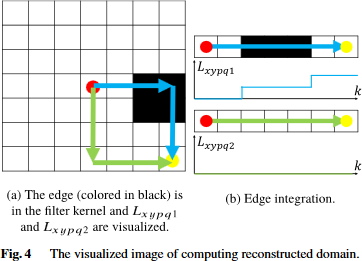I’m not sure what is it your asking, but to find the distance between two pixels, you could use the hypotenuse route which is very easy. sqrt(x_length^2+y_length^2)

Now, if you’re asking to find the distance taking into account of pixels as blocks, that’s a bit more complicated as it’s not straight-forward as the hypotenuse route.

I would like you to clarify on this.

In Fig. 4, there are two routes, Lxypq1 is right and then down. If the range is [0,1], and the fig. only has white and black pixels, Lxypq1=2 because it encounters 2 edges. This can be calculated from the differences of every step added together: abs(0-1)+abs(1-2)+abs(2-3)+abs(3-4)+abs(4-5)+abs(5-6), the numbers being the ith pixel along the path, at least that is how I think of it. I am just having trouble seeing it in (G’MIC) code since I am not a programmer.

Hold on, I think I actually start to understand what you want. You want to calculate the number of step it takes to get from one distance to another per axis, and blocks means 0?

It is simpler than you think. If I move from red dot one pixel, is there a change? No. Next pixel there is a change (or distance) of -1 but |-1|=1, etc. In total, there are two changes of 1, so Lxypq1=2. In the graph, there are two steps.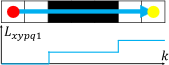What I have so far, with my super poor coding skills, is

``````foo_d:
(1,1,0,0;1,1,0,0;1,1,1,1;1,1,1,1) (0)
f. "
for(
k=0,k<w#0,
abs(i(#0,k++,0)-i(#0,k+++1,0))+
abs(i(#0,w-1,k++)-i(#0,w-1,k+++1))
)
"
``````

but I get L=1, which is incorrect.

Edit I am not looping properly. It looks like the calculations are inheriting the last values of the row and column.

I was thinking more of this.

``````f "
begin(
x_length=4;
y_length=4;
line_a_x(v)=(
val=I(x,y,z,0,2);
changes=0;
for(n=1,n<v-1,n++,
if(I(x+n,y,z,0,2)!=val,
changes++;
);
);
changes;
);
);
line_a_x(x_length);
"
``````

All you need to do is to add more macros, and then you have what you want.

Never mind. Thought of a way to minimize loop to find objects centers

Could someone explain how pattern works? E.g., `polygon` uses pattern.

``````polygon (+):
N>=1,x1[%],y1[%],...,xN[%],yN[%],_opacity,_pattern,_color1,...
``````

Pattern is an hexadecimal number with 8 digits, like 0xCCCCFFFF.
It has then 64 bits (0 or 1) that defines the pattern uses to draw the outline of the polygon.
Commonly used patterns are:

• `0xFFFFFFFF` : plain line. Use it to draw an outlined polygon.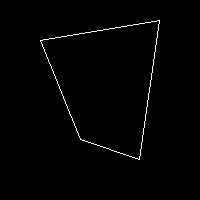• `0xF0F0F0F0` : dashed line with 8px plain, 8px empty: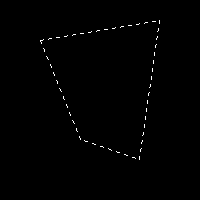• `0xCCCCCCCC` : dashed line with 2px plain, 2px empty: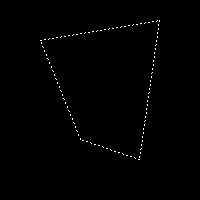• `0xAAAAAAAA`: dashed line with 1px plain, 1px empty: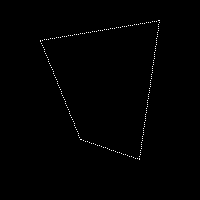It’s quite flexible, as you can choose exactly what pattern you want.
For instance:

• `0xF99FF99F` gives something like this: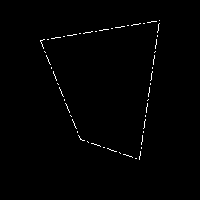Sorry, I have no idea how to decipher the hexadecimal value and how it contributes to the pattern.Could you please elaborate?

(Looks like more than 8 digits `0xccccccccc` produces a solid line.)

You just write the sequence of `1` and `0` that defines your pattern (64 digits), and then use a binary to hexadecimal converter, as: https://www.rapidtables.com/convert/number/binary-to-hex.html1 Like